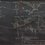# Polylogarithm recursion identity

I recently discovered a recursive identity for $$\mathrm{Li}_{-m}(z)$$ for $$m$$ a positive integer. Hopefully this will be of some use when tackling problems seeking for the value of some polylogarithmic sum.

$\mathrm{Li}_{-m}(z) = \frac{z}{1-z} \left[ 1+\sum_{k=0}^{m-1} \binom{m}{k} \mathrm{Li}_{-k}(z) \right]$

Proof:

Consider $$\displaystyle \sum_{n=1}^{\infty} z^n(n+1)^m$$. We have

\begin{align} \sum_{n=1}^{\infty} z^n(n+1)^m &= \sum_{n=1}^{\infty} z^n \sum_{k=0}^m \binom{m}{k} n^k \\ &= \sum_{n=1}^{\infty} z^nn^m + \sum_{k=0}^{m-1} \binom{m}{k} \sum_{n=1}^{\infty} z^nn^k \\ &= \mathrm{Li}_{-m}(z) + \sum_{k=0}^{m-1} \binom{m}{k} \mathrm{Li}_{-k}(z) \end{align}

We also have

\begin{align} \sum_{n=1}^{\infty} z^n(n+1)^m &= \frac{1}{z} \sum_{n=2}^{\infty} z^nn^m \\ &= \frac{1}{z} \mathrm{Li}_{-m}(z)-1 \end{align}

Equating the two gives

$\mathrm{Li}_{-m}(z) + \sum_{k=0}^{m-1} \binom{m}{k} \mathrm{Li}_{-k}(z) = \frac{1}{z} \mathrm{Li}_{-m}(z)-1$

When rearranged, we obtain

$\mathrm{Li}_{-m}(z) = \frac{z}{1-z} \left[ 1+\sum_{k=0}^{m-1} \binom{m}{k} \mathrm{Li}_{-k}(z) \right]$

as required.Note by Jake Lai
3 years, 6 months ago

MarkdownAppears as
*italics* or _italics_ italics
**bold** or __bold__ bold
- bulleted- list
• bulleted
• list
1. numbered2. list
1. numbered
2. list
Note: you must add a full line of space before and after lists for them to show up correctly
paragraph 1paragraph 2

paragraph 1

paragraph 2

[example link](https://brilliant.org)example link
> This is a quote
This is a quote
    # I indented these lines
# 4 spaces, and now they show
# up as a code block.

print "hello world"
# I indented these lines
# 4 spaces, and now they show
# up as a code block.

print "hello world"
MathAppears as
Remember to wrap math in $$...$$ or $...$ to ensure proper formatting.
2 \times 3 $$2 \times 3$$
2^{34} $$2^{34}$$
a_{i-1} $$a_{i-1}$$
\frac{2}{3} $$\frac{2}{3}$$
\sqrt{2} $$\sqrt{2}$$
\sum_{i=1}^3 $$\sum_{i=1}^3$$
\sin \theta $$\sin \theta$$
\boxed{123} $$\boxed{123}$$

## Comments

Sort by:

Top Newest

Similarly $\mathrm{Li}_{-m}(z)=\frac{\left(-1\right)^m}{1-z}\left[\sum _{k=0}^{m-1}\left(-1\right)^k\binom{m}{k}\mathrm{Li}_{-k}(z)\right]$

- 3 years, 6 months ago

Log in to reply

Nice!

- 3 years, 6 months ago

Log in to reply

A point to note is that $$\operatorname{Li}_{-m} (z)$$ , when $$m$$ is a positive integer, already has a closed form in terms of rational polynomial functions.

- 3 years ago

Log in to reply

×

Problem Loading...

Note Loading...

Set Loading...